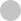#### Progress

0%#### Basic Data Structures#### Overview

•Vanilla Binary Search
•Finding Boundary

#### Sorted Array

•First Element Not Smaller Than Target 🔒
•First Occurrence
•Square Root 🔒

#### Implicitly Sorted Array#### Introduction

•Recursion Review
•DFS Fundamentals

#### Combinatorial Search

•DFS with States
•Backtracking Fundamentals
•Permutations
•Letter Combinations of Phone Number 🔒

#### Memoization

•Memoization Intro 🔒
•Word Break 🔒
•Decode Ways 🔒#### Introduction

•BFS Fundamentals

#### BFS on Tree#### Introduction

•Graph Fundamentals
•BFS on Graphs 🔒
•DFS on Graph 🔒
•BFS or DFS 🔒
•Matrix as Graph 🔒

#### BFS

•Shortest Path 🔒
•Word Ladder 🔒

#### Matrix as Graph

•Flood Fill 🔒
•Number of Islands 🔒
•Knight Minimum Moves 🔒

#### Directed Graph

•Course Schedule 🔒#### Same Direction

•Remove Duplicates 🔒
•Middle of Linked List 🔒
•Move Zeros 🔒

#### Opposite Direction

•Two Sum Sorted 🔒
•Valid Palindrome 🔒
•Trapping Rain Water 🔒

#### Prefix Sum

•Subarray Sum 🔒
•Subarray Sum Divisible by K 🔒#### Introduction

•Heap Fundamentals

#### Top K

•K Closest points 🔒
•Merge K Sorted Lists 🔒#### Binary Search#### Sequence

•House Robber 🔒
•Coin Change 🔒

#### Grid

•Number of Robot Paths 🔒
•Minimal Path Sum 🔒
•Maximal Square 🔒

#### Two Sequences

•Edit Distance 🔒
•Longest Common Subsequence 🔒

#### Game Theory

•Divisor Game 🔒#### Monotonic Stack

•Sliding Window Maximum

#### Data Structure Design

•LRU Cache 🔒# Types of Dynamic Programming Questions

Here's the breakdown. We also highlighted the keywords that indicate it's likely a dynamic programming problem.

## Sequence

This is the most common type of DP problem and a good place to get a feel of dynamic programming. In the recurrence relation,`dp[i]` normally means max/min/best value for the sequence ending at index i.

• House robber - find maximum amount of loot
• Coin change - find minimum amount of coins needed to make up an amount

## Grid

This is the 2D version of the sequence DP. `dp[i][j]` means max/min/best value for matrix cell ending at index i, j.

• Robot unique paths - number of ways for robot to move from top left to bottom right
• Min path sum - find path in a grid with minimum cost
• Maximal square - find maximal square of 1s in a grid of 0s and 1s

## Dynamic number of subproblems

This is similar to "Sequence DP" except `dp[i]` depends on a dynamic number of subproblems, e.g. `dp[i] = max(d[j]..) for j from 0 to i`.

• Longest Increasing Subsequence - find the longest increasing subsequence of an array of numbers
• Buy/sell stock with at most K transactions - maximize profit by buying and selling stocks using at most K transaction

## Partition

This is a continuation of DFS + memoization problems. These problems are easier to reason and solve with a top-down approach. The key to solve these problems is to draw the state-space tree and then traverse it.

• Decode ways - how many ways to decode a string
• Word break - partition a word into words in a dictionary
• Triangle - find the smallest sum path to traverse a triangle of numbers from top to bottom
• Partition to Equal Sum Subsets - partition a set of numbers into two equal-sum subsets

## Two Sequences

This type of problem has two sequences in their problem statement. `dp[i][j]` represents the max/min/best value for the first sequence ending in index i and second sequence ending in index j.

• Edit distance - find the minimum distance to edit one string to another
• Longest common subsequence - find the longest common subsequence that is common in two sequences

## Game theory

This type of problem asks for whether a player can win a decision game. The key to solving game theory problems is to identify winning state, and formulating a winning state as a state that returns a losing state to the opponent

• Coins in a line
• Divisor game
• Stone game